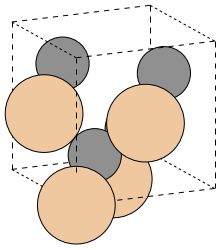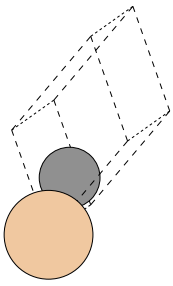# Primitive unit cells¶

Understanding the definitions of the crystal structure are of paramount importance. If your structure is wrong your results are wrong. Thus it is highly recommended to check your data carefully before even starting the calculation.

This tutorial shows how to generate the structure and how to check its relationship with the primitive unit cell and how to generate primitive unit cells with various definitions.

In :
# Import the basic libraries

# ASE system
import ase
from ase import Atom, Atoms
from ase import io
from ase.lattice.spacegroup import crystal

# Spacegroup/symmetry library
from pyspglib import spglib

# The qe-util package
from qeutil import QuantumEspresso

# iPython utility function
from IPython.core.display import Image

# Configure qe-util for local execution of the Quantum Espresso on four processors
QuantumEspresso.pw_cmd='mpiexec -n 4 pw.x < %(infile)s > %(outfile)s'


## Crystal definition¶

Here we define a $\beta$-SiC crystal: a cubic zincblende crystal with a spacegroup F-43m (space group number 216) and an experimental lattice constant (here, A=4.3596 A). The atomic positions are specified in the fractional (crystallographic) coordinates; i.e. coordinates measured in units of lattice constants in the coordinate system defined by lattice vectors. The unit cell is specified as three lengths (in angstrom) of the lattice vectors and three angles (in degrees) between these vectors. The crystal axis are oriented in the conventional way in the Cartesian (X,Y,Z) coordinate system:

• Vector $\vec{A}$ is along the X axis
• Vector $\vec{B}$ is in the XY plane

This crystal is defined in what is usually called Conventional Unit Cell.

In :
a=4.3596                                         # Lattice constant in Angstrom
cryst = crystal(['Si', 'C'],                     # Atoms in the crystal
[(0, 0, 0), (0.25, 0.25, 0.25)], # Atomic positions (fractional coordinates)
spacegroup=216,                  # International number of the spacegroup of the crystal
cellpar=[a, a, a, 90, 90, 90])   # Unit cell (a, b, c, alpha, beta, gamma) in Angstrom, Degrees


We can display the picture of the crystal. The ase.io.write procedure, used here, is a very flexible tool. It can write the crystal in numerous formats, it can even run external tools for rendering a 3D scene. Here, we just use a simple renderer build into the write procedure to store a picture of the crystal into a disk file and then to display it.

In :
# Write the image to disk file
ase.io.write('crystal.png',       # The file where the picture get stored
cryst,               # The object holding the crystal definition
format='png',        # Format of the file
show_unit_cell=2,    # Draw the unit cell boundaries
rotation='115y,15x', # Rotate the scene by 115deg around Y axis and 15deg around X axis
scale=35)            # Scale of the picture

# Display the image
Image(filename='crystal.png')

Out:Most of the calculations are done on the primitive unit cell of the crystal (instead of the traditional, crystallographic unit cell). The primitive unit cell is usually substantially smaller, thus the calculations are much faster. The spglib library contains also a function which can identify a primitive unit cell for a given structure. In this part of the tutorial we create a primitive unit cell for the crystal. Usually we do not need to do it explicitly - the qeutil library extracts this information internally when it is needed. Still it is worthwhile to do it by hand to learn about relationships. Furthermore one needs to note that the definition of the primitive unit cell is not unique. The same parallerogram in space may be described by many different base vectors - depending on selection of edges used for the base vectors.

The spglib.find_primitive function returns three arrays containing respectively (remember that lists and array indexes start from 0 in python):

• unit cell vectors (3x3) matrix (in Angstrom)
• atomic positions (3xN-atoms) matrix (fractional coordinates)
• list of atomic numbers of the atoms in the unit cell

The returned primitive unit cell is usually not the one which is conventionally used in the literature (e.g. it usually differs from the one required by the Quantum Espresso). If you want some particular form of the unit cell you have to do the conversion yourself (see below).

Note 1: The primitive unit cell is defined in the Cartesian coordinate system (as a 3x3 matrix of lattice vectors coordinates in angstroms). It corresponds to the orientation of the crystal for which it was derived. The orientation of both crystals are the same but the primitive unit cell is usually not oriented in the conventional way (see the remark about orientation of axis above). Particularly in the case presented here the A, B, C axes of the primitive lattice are oriented, respectively, in the XY, XZ and XY planes (see the matrix below).

Note 2: The atomic coordinates below are specified in fractional coordinates in the primitive unit cell. Thus they are different then the fractional coordinatesin the conventional unit cell defined above. This is an important rule: The fractional coordinates are always defined and measured by the vectors of the current unit cell.

In :
# Find a primitive cell of the structure
puc=spglib.find_primitive(cryst)

# Parameters of the unit cell
print 'Primitive unit cell (Angstrom, carthesian coordinates):\n', puc
print '\nAtomic positions (fractional, crystalographic):\n', puc

Primitive unit cell (Angstrom, carthesian coordinates):
[[ 2.1798 -2.1798  0.    ]
[-2.1798 -0.     -2.1798]
[ 2.1798  2.1798  0.    ]]

Atomic positions (fractional, crystalographic):
[[  1.38777878e-17   5.55111512e-17   2.77555756e-17]
[ -2.50000000e-01  -5.00000000e-01   2.50000000e-01]]


Based on the parameters of the primitive unit cell we can build a crystal object for it. Just feed the parameters stored in puc variable to the creator of the generic atomic systems: Atoms. Then we will check the symmetry of the created structure to verify it is the same.

In :
# Create the primitive cell crystal
cryst_prim=Atoms(                         # Create a generic atomic structure
cell=puc,             # Unit cell (Angstrom)
scaled_positions=puc, # Atomic positions (fractional)
numbers=puc,          # Atomic numbers
pbc=True)                # Use Periodic Boundary Conditions

In :
# Check if the symmetry is still the same
print spglib.get_spacegroup(cryst_prim)

F-43m      (216)


For the FCC structure we have here the traditional primitive unit cell is quite different from the one obtained above. Typically the vectors of the primitive unit cell of the FCC structure point to the centers of faces of three sides of the cube adjanced to the origin giving the following cell matrix: $$\frac{a}{2}\left[ \begin{array}{ccc} 1 & 0 & 1 \\ 1 & 1 & 0 \\ 0 & 1 & 1 \end{array}\right]$$

One can transform the unit cell created above (cryst_prim) into this standard prymitive unit cell.

The trick is simple: build a new unit cell with the same cartesian atomic positions and your desired unit cell. The creation procedure will automatically fold the atoms into correct positions inside the unit cell.

In :
# define the new unit cell
new_uc=a*array([[1,0,1],[1,1,0],[0,1,1]])/2

print "New primitive unit cell (A)\n", new_uc

New primitive unit cell (A)
[[ 2.1798  0.      2.1798]
[ 2.1798  2.1798  0.    ]
[ 0.      2.1798  2.1798]]

In :
# Create a new crystal with a new primitive unit cell
new_crystal=Atoms(cell=new_uc,                               # Define new unit cell
positions=cryst_prim.get_positions(),    # Put atoms in cartesian positions from the cryst_prim
numbers=cryst_prim.get_atomic_numbers(),         # Set atomic numbers
pbc=True)                                # Make the structure periodic

print "Atomic positions (fractional)\n", new_crystal.get_scaled_positions()

Atomic positions (fractional)
[[  0.00000000e+00   2.77555756e-17   0.00000000e+00]
[  2.50000000e-01   2.50000000e-01   2.50000000e-01]]

In :
# Take a look, same orientation as before
ase.io.write('crystal-prim.png',new_crystal,format='png',show_unit_cell=2, rotation='115y,15x', scale=40)
Image(filename='crystal-prim.png')

Out:The image above shows exactly the type of the primitive unit cell we have requested. Furthermore, if you compare the orientation of the atoms here and on the picture of the conventional unit cell you will notice that they are the same. The whole procedure just "cut out" the primitive unit cell out of the conventional one. Note the fractional coordinates of the carbon atom in two definitions of the primitive unit cell. In the first it is [-1/4, 1/2, 1/4] while in the second one it is [1/4, 1/4, 1/4] as expected.

In :